Examples

Chapter 7 Class 11 Binomial Theorem
Serial order wise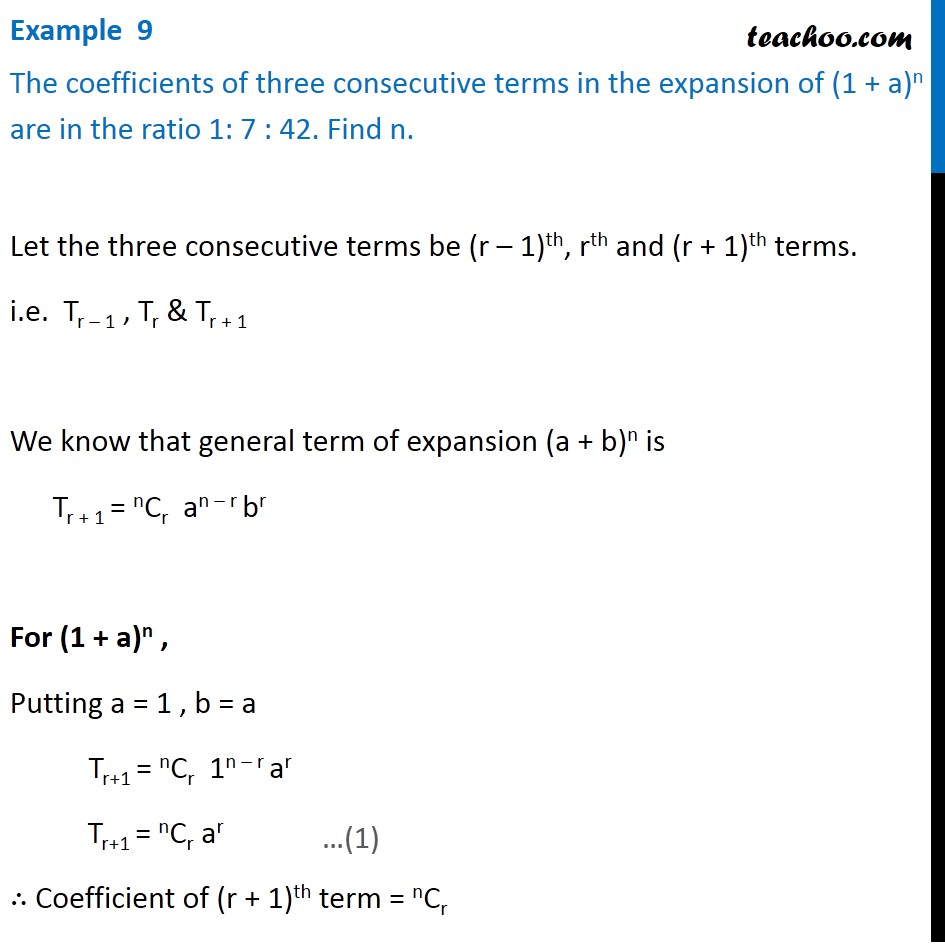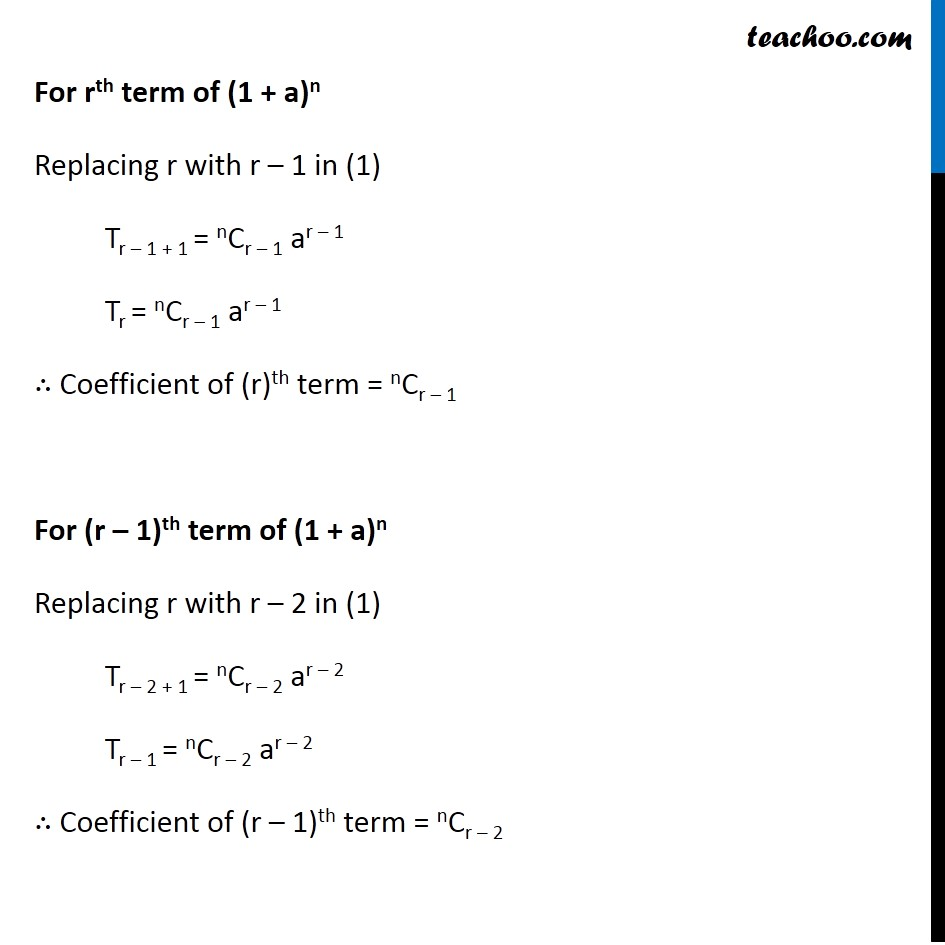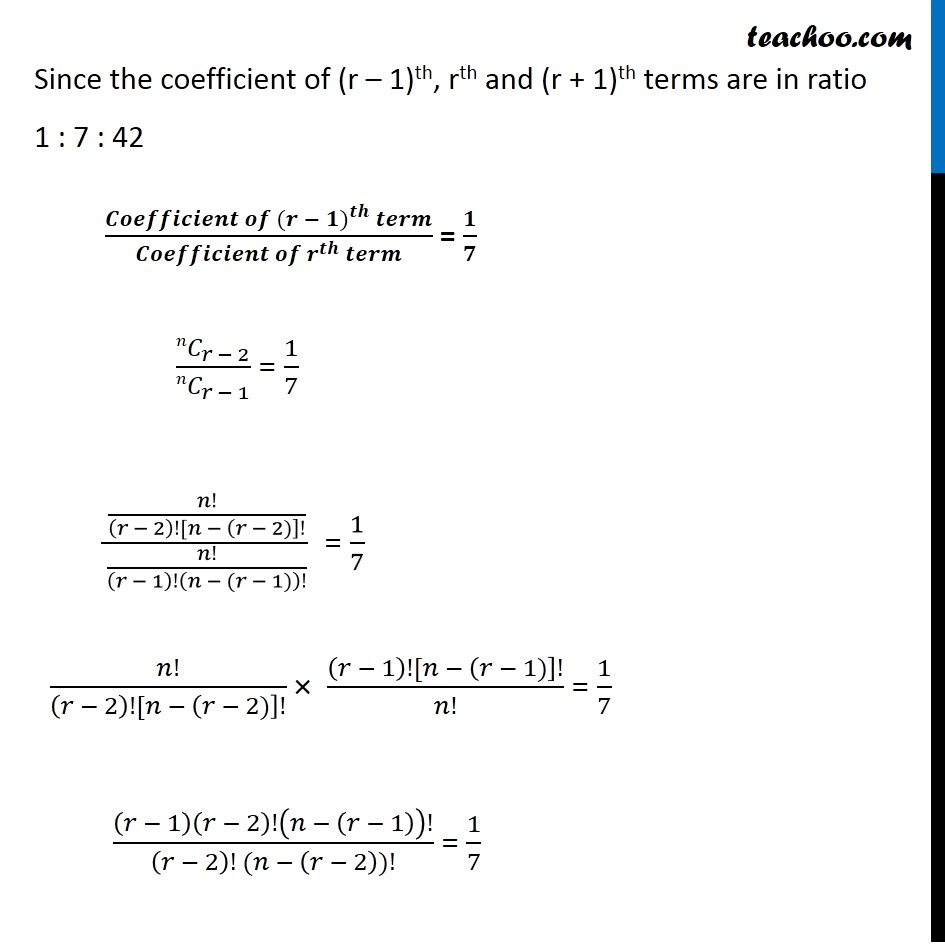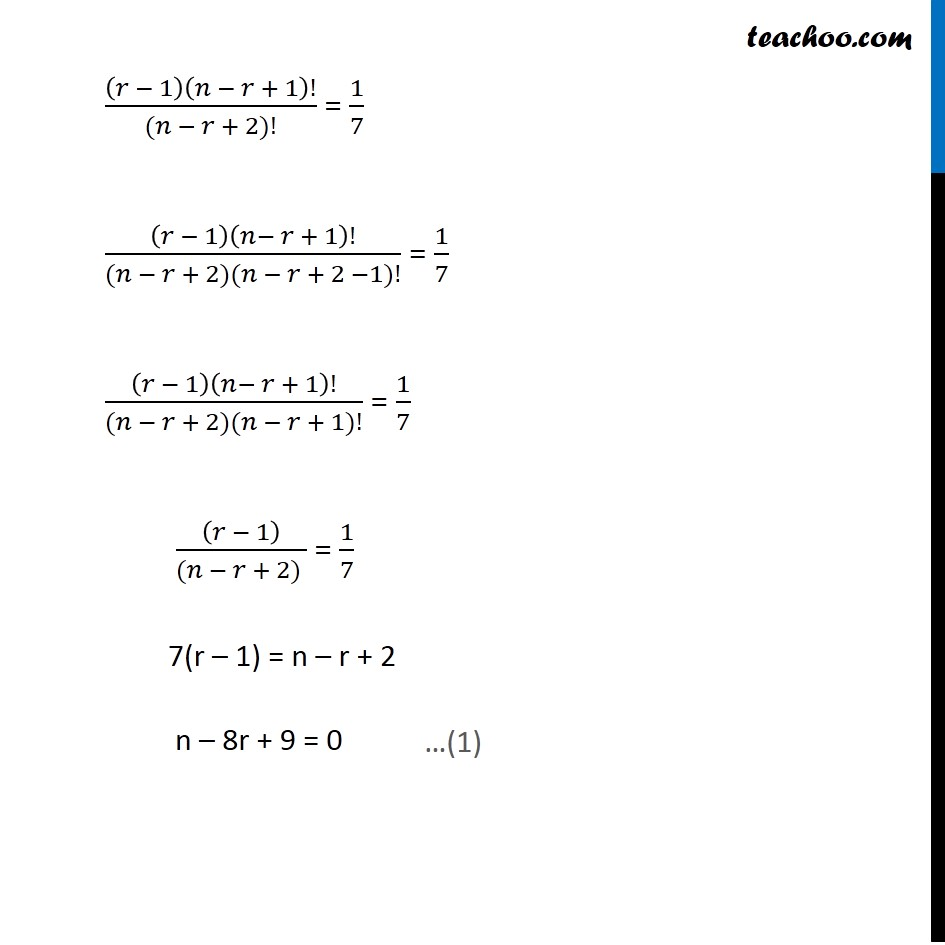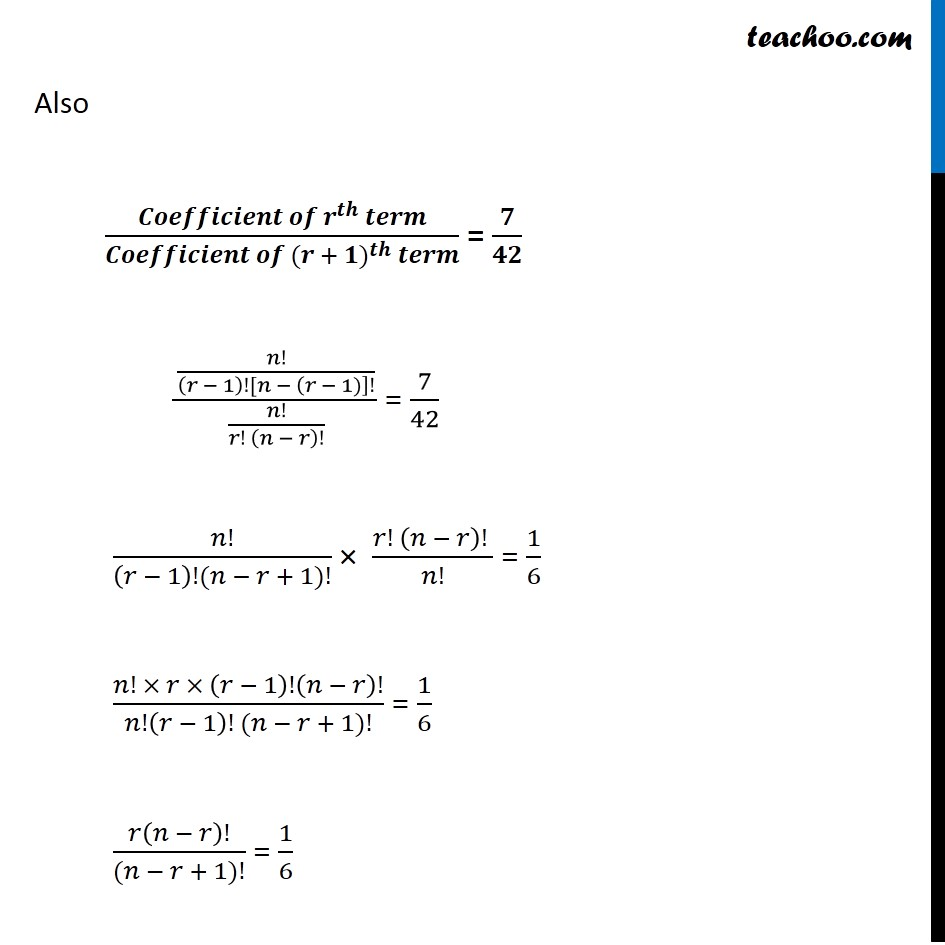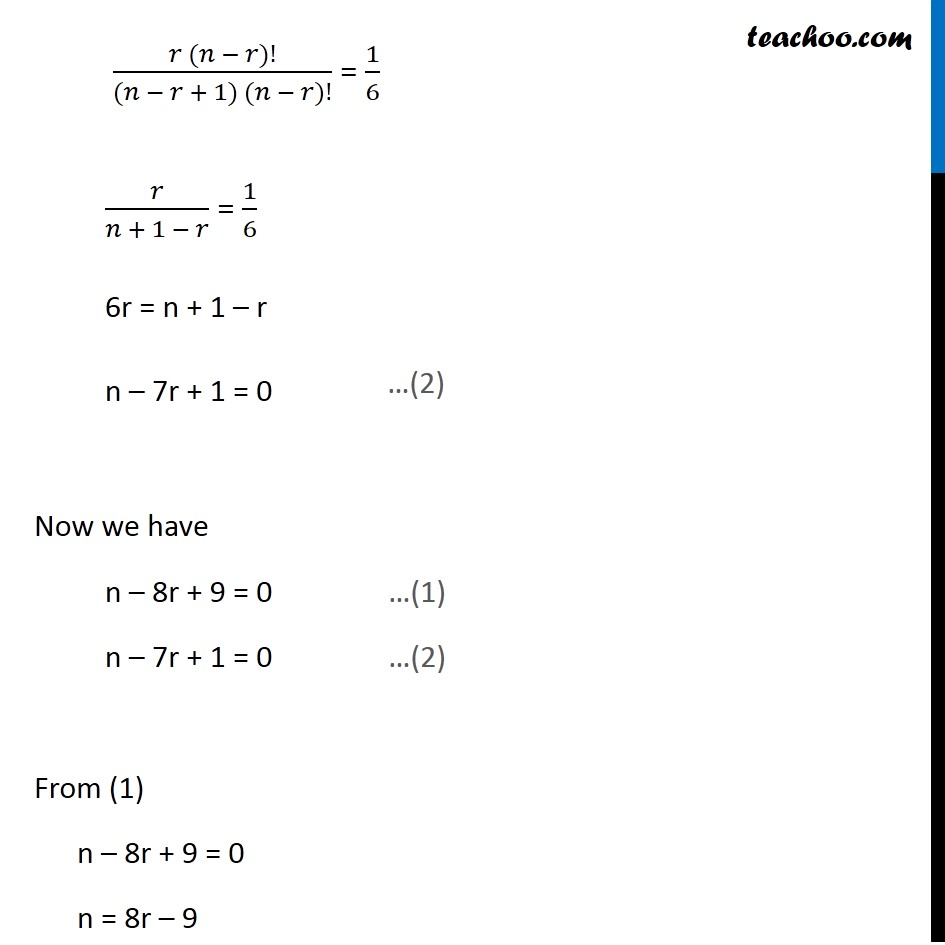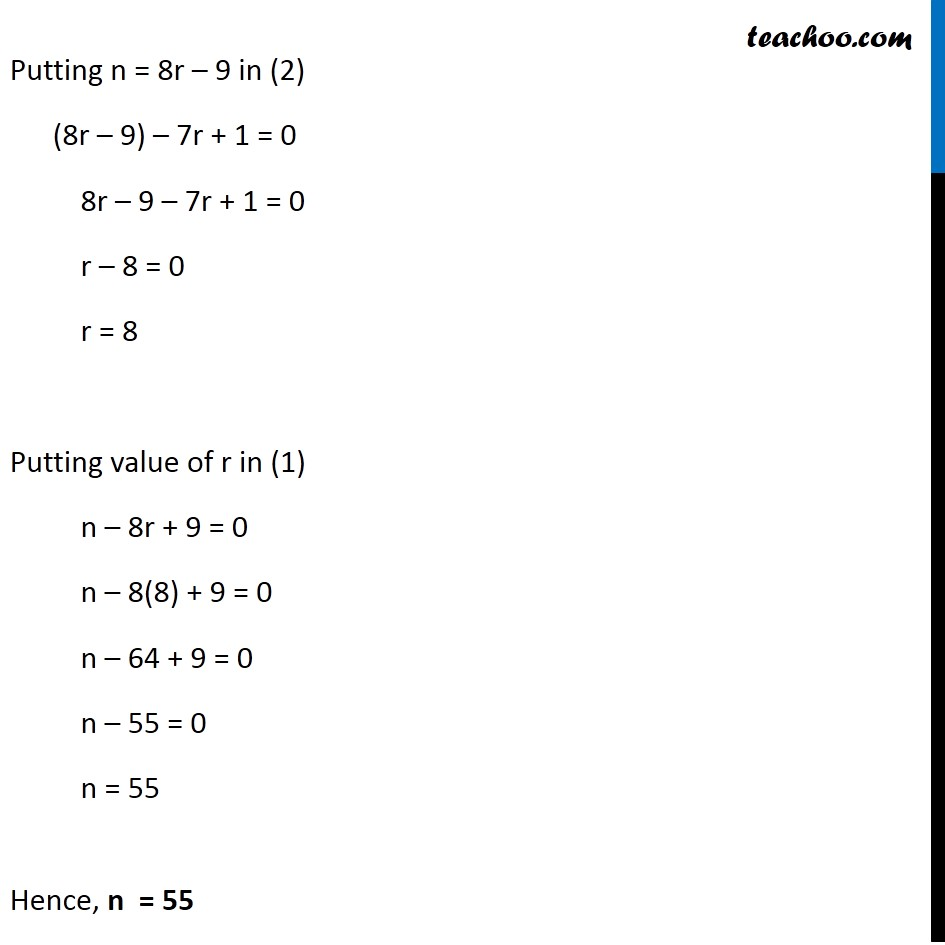Learn in your speed, with individual attention - Teachoo Maths 1-on-1 Class

### Transcript

Question 5 The coefficients of three consecutive terms in the expansion of (1 + a)n are in the ratio 1: 7 : 42. Find n. Let the three consecutive terms be (r – 1)th, rth and (r + 1)th terms. i.e. Tr – 1 , Tr & Tr + 1 We know that general term of expansion (a + b)n is Tr + 1 = nCr an – r br For (1 + a)n , Putting a = 1 , b = a Tr+1 = nCr 1n – r ar Tr+1 = nCr ar ∴ Coefficient of (r + 1)th term = nCr For rth term of (1 + a)n Replacing r with r – 1 in (1) Tr – 1 + 1 = nCr – 1 ar – 1 Tr = nCr – 1 ar – 1 ∴ Coefficient of (r)th term = nCr – 1 For (r – 1)th term of (1 + a)n Replacing r with r – 2 in (1) Tr – 2 + 1 = nCr – 2 ar – 2 Tr – 1 = nCr – 2 ar – 2 ∴ Coefficient of (r – 1)th term = nCr – 2 Since the coefficient of (r – 1)th, rth and (r + 1)th terms are in ratio 1 : 7 : 42 (𝑪𝒐𝒆𝒇𝒇𝒊𝒄𝒊𝒆𝒏𝒕 𝒐𝒇 〖(𝒓 − 𝟏)〗^𝒕𝒉 𝒕𝒆𝒓𝒎)/(𝑪𝒐𝒆𝒇𝒇𝒊𝒄𝒊𝒆𝒏𝒕 𝒐𝒇 𝒓^𝒕𝒉 𝒕𝒆𝒓𝒎) = 𝟏/𝟕 〖𝑛𝐶〗_(𝑟 − 2)/〖𝑛𝐶〗_(𝑟 − 1) = 1/7 (𝑛!/((𝑟 − 2)![𝑛 − (𝑟 − 2)]!))/(𝑛!/(𝑟 − 1)!(𝑛 − (𝑟 − 1))!) = 1/7 𝑛!/((𝑟 − 2)![𝑛 − (𝑟 − 2)]!) × ((𝑟 − 1)![𝑛 − (𝑟 − 1)]!)/𝑛! = 1/7 (𝑟 − 1)(𝑟 − 2)!(𝑛 − (𝑟 − 1))!/((𝑟 − 2)! (𝑛 − (𝑟 − 2))!) = 1/7 (𝑟 − 1)(𝑛 − 𝑟 + 1)!/((𝑛 − 𝑟 + 2)!) = 1/7 (𝑟 − 1)(𝑛− 𝑟 + 1)!/((𝑛 − 𝑟 + 2)(𝑛 − 𝑟 + 2 −1)!) = 1/7 (𝑟 − 1)(𝑛− 𝑟 + 1)!/((𝑛 − 𝑟 + 2)(𝑛 − 𝑟 + 1)!) = 1/7 ((𝑟 − 1))/((𝑛 − 𝑟 + 2) ) = 1/7 7(r – 1) = n – r + 2 n – 8r + 9 = 0 Also (𝑪𝒐𝒆𝒇𝒇𝒊𝒄𝒊𝒆𝒏𝒕 𝒐𝒇 𝒓^𝒕𝒉 𝒕𝒆𝒓𝒎)/(𝑪𝒐𝒆𝒇𝒇𝒊𝒄𝒊𝒆𝒏𝒕 𝒐𝒇 〖(𝒓 + 𝟏)〗^𝒕𝒉 𝒕𝒆𝒓𝒎) = 𝟕/𝟒𝟐 (𝑛!/((𝑟 − 1)![𝑛 − (𝑟 − 1)]!))/(𝑛!/(𝑟! (𝑛 − 𝑟)!)) = 7/42 𝑛!/((𝑟 − 1)!(𝑛 − 𝑟 + 1)!) × (𝑟! (𝑛 − 𝑟)! )/𝑛! = 1/6 (𝑛! × 𝑟 × (𝑟 − 1)!(𝑛 − 𝑟)!)/(𝑛!(𝑟 − 1)! (𝑛 − 𝑟 + 1)!) = 1/6 𝑟(𝑛 − 𝑟)!/((𝑛 − 𝑟 + 1)!) = 1/6 (𝑟 (𝑛 − 𝑟)!)/((𝑛 − 𝑟 + 1) (𝑛 − 𝑟)!) = 1/6 𝑟/(𝑛 + 1 − 𝑟) = 1/6 6r = n + 1 – r n – 7r + 1 = 0 Now we have n – 8r + 9 = 0 …(1) n – 7r + 1 = 0 …(2) From (1) n – 8r + 9 = 0 n = 8r – 9 Putting n = 8r – 9 in (2) (8r – 9) – 7r + 1 = 0 8r – 9 – 7r + 1 = 0 r – 8 = 0 r = 8 Putting value of r in (1) n – 8r + 9 = 0 n – 8(8) + 9 = 0 n – 64 + 9 = 0 n – 55 = 0 n = 55 Hence, n = 55